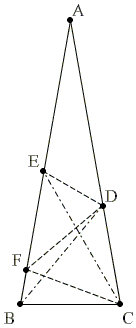# The 80-80-20 Triangle Problem, Solution #5Let ABC be an isosceles triangle (AB = AC) with ∠BAC = 20°. Point D is on side AC such that ∠CBD = 50°. Point E is on side AB such that ∠BCE = 60°. Find the measure of ∠CED.

Solution

This is Solution 4 from [Knop].

Let F be on AB with ∠BCF = 20°.Then ∠BFC = 80° = ∠ABC, so that ΔBCF is isosceles and CF = BC.

Since also CD = BC and ∠DCF = 60°, ΔCDF is equilateral and, in particular, DF = CF.

ΔCEF is isosceles because ∠CEF = ∠ECF = 40°. Therefore, DF = EF. It follows that F is equidistant from C, D, and E such that it serves the circumcenter of ΔCDE. ∠CED is inscribed into a circle with center F and is subtended by the same arc as the central angle CFD. But the latter is 60°. Therefore, ∠CED = 30°.

### Reference

1. C. Knop, Nine Solutions to One Problem, Kvant, 1993, no 6.### The 80-80-20 Triangle Problem